Monte-Carlo methods for partial differential equations

Deterministic partial differential equations can be solved numerically by probabilistic algorithms such as Monte-Carlo methods, stochastic particle methods, ergodic algorithms, etc. (cf. also Differential equation, partial).

The simplest example is the heat equation in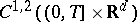: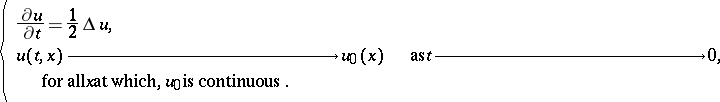For a large class of functions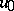, the unique solution is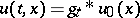, whereis the heat kernel. Thus,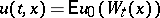, where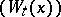is any Brownian motion such that, almost surely. The strong law of large numbers applies: if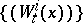are independent copies of, one has, almost surely,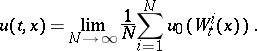A Monte-Carlo procedure to compute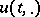at a single pointconsists in the simulation ofindependent copies of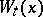and the computation of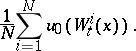This procedure requires the simulation ofindependent real-valued Gaussian random variables of meanand variance(cf. Random variable; Gaussian process). The convergence rate of the procedure is described by fluctuation results, large-deviation estimates, etc. (cf. also Probability of large deviations). The most elementary estimate concerns the standard deviation of the random error:More generally, letbe an integro-differential operator (cf. also Integro-differential equation), and consider the evolution problemFrom now on, it is assumed that there is a-valued Markov processsuch that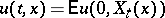(for a discussion on the assumptions thatmust satisfy, see [a3], Chap. XIII, Sect. 3). Then, if it is possible to simulate independent copies of the process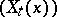, one can approximate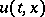byFor example, in neutronics, the process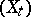is the pair (position,velocity), and the velocity is a pure jump process (in this case,is a transport operator) whose simulation is simple: one simulates the times at which the velocity changes and the new velocity after each change time independently (the change times have an exponential law).

Most often, the law ofcannot be simulated exactly, but it may be possible to approximate it suitably. For example, whenis the solution of a stochastic differential equation driven by a Brownian motion (in this case,is a second-order differential operator), one can discretize the stochastic differential equation in time. The simplest discretization scheme (the Euler scheme) defines an approximate processdepending on the discretization step, whose simulation requires the simulation of independent Gaussian random variables only. The approximation formula is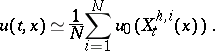The global error of this procedure can be decomposed as the sum of(the discretization error) and of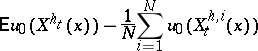(the statistical error). Under appropriate assumptions, the discretization error can be expanded in terms of.

The numerical procedure can be adapted to the solution of problems in bounded domains. Dirichlet boundary conditions lead to the simulation of processes stopped at the boundary. The simulation of reflected diffusion processes (cf. Diffusion process) solves parabolic or elliptic partial differential equations with suitable Neumann boundary conditions.

Letbe the formal adjoint of. Note that the probability distribution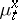of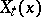is a solution to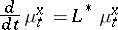in the sense of distributions (cf. Generalized function). The solutioncan be approximated by the empirical distribution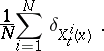This procedure is used in neutronics.

To certain non-linear partial differential equations one can associate processeswhich are not Markovian, but are such that the pairs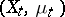are Markovian, where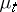is the probability law of the random variable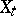. Besides, in some cases, the law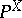of the processcan be described by means of the theory of propagation of chaos (see [a4] and the references therein). That means that the lawis the limit in probability, whengoes to infinity, of the empirical distributions of the paths ofparticles in a mean field interaction. The interaction between the particles is related to the non-linear differential operator of the partial differential equation under consideration. If one can simulate the stochastic particle system, then an algorithm can be built from the formulawhereare the locations at timeof the interacting particles. For example, consider an interaction kernel, a family ofindependent Brownian motions, and the system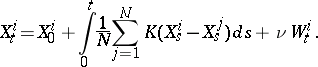The time discretization of such systems permits one to approximate equations such as Vlasov–Poisson–Fokker–Planck equations (cf. Vlasov–Poisson–Fokker–Planck system), the viscous Burgers equation (cf. Diffusion equation; Turbulence, mathematical problems in), the incompressible Navier–Stokes equation for the vorticity in(cf. Navier–Stokes equations), etc. In the latter case,is the Biot–Savart kernel, and the algorithm is the Chorin random vortex method (see [a2] for numerical considerations). Similarly, the simulation of interacting branching processes (cf. Branching process) permits one to approximate the solutions of convection-reaction-diffusion equations. One difficulty of the mathematical study of these methods is to show that the statistical errors (on the distribution function of, e.g.) have standard deviations of order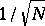although the particles are dependent. For theoretical aspects related to probabilistic algorithms for partial differential equations, including the Boltzmann equation, see, for instance, [a1] and the references therein.